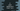# 5 different C programs to print all natural numbers from 1 to n## C program to print all natural numbers from 1 to n:

Write a C program to print all natural numbers from 1 to n. To print the sequence of natural numbers, we have to use a loop. We can use any loop to print the natural numbers in a given range.

In this post, we will learn how to use different loops to print the natural numbers in a range. The program will take the value of n as an input from the user and print all natural numbers from 1 to n on the console.

### Algorithm to follow:

We will use the below algorithm:

• Get the value of n as an input from the user.
• Run a loop from 1 to n.
• On each iteration of the loop, print the current value.

### Method 1: By using a for loop:

Let’s use a for loop to solve this program.

``````#include <stdio.h>

int main()
{
int n;

printf("Enter the value of n: ");
scanf("%d", &n);

for (int i = 1; i <= n; i++)
{
printf("%d ", i);
}
printf("\n");
}``````

Here,

• n is an integer variable to hold the upper range.
• It asks the user to enter the value of n and uses scanf to read it to variable n.
• The for loop starts from i = 1 and ends at i = n.
• Inside the loop, we are printing the value of i.

If you run the above program, it will give output as like below:

``````Enter the value of n: 10
1 2 3 4 5 6 7 8 9 10

Enter the value of n: 20
1 2 3 4 5 6 7 8 9 10 11 12 13 14 15 16 17 18 19 20``````

### Method 2: By using a for loop with a separate method:

Let’s use a separate method to print the numbers. We can move the code that prints the value of i to a different method.

``````#include <stdio.h>

void printNumbers(int n)
{
for (int i = 1; i <= n; i++)
{
printf("%d ", i);
}
printf("\n");
}

int main()
{
int n;

printf("Enter the value of n: ");
scanf("%d", &n);

printNumbers(n);
}``````

In this program, we created a new method printNumbers. This method takes the value of n as its argument. The return type of this method is void i.e. it doesn’t return anything.

Inside the method, we are using a for loop to print the values of i from 1 to n. Once the loop ends, we are printing a new line.

This is similar to the previous program. The only difference is that we moved the code to a new method and this method is called from main.

It will give a similar result.

### Method 3: By using a while loop:

We can also use a while loop to write the same program. The difference is that while loop will initialize the variable before it use it in the loop.

``````#include <stdio.h>

void printNumbers(int n)
{
int i = 1;

while (i <= n)
{
printf("%d ", i);
i++;
}
printf("\n");
}

int main()
{
int n;

printf("Enter the value of n: ");
scanf("%d", &n);

printNumbers(n);
}``````

This is almost similar to the previous example program. We are changing the printNumbers method to use while loop instead of for loop.

It will print similar output.

### Method 4: By using do-while loop:

We can also use a do-while loop to do the same process. The difference between a while loop and do-while loop is that while loop checks the condition and runs the code in it but do-while loop runs the code and checks the condition.

Let me show you how to write the same program using a do-while loop:

``````#include <stdio.h>

void printNumbers(int n)
{
int i = 1;

do
{
printf("%d ", i);
i++;
}while(i < n + 1);

printf("\n");
}

int main()
{
int n;

printf("Enter the value of n: ");
scanf("%d", &n);

printNumbers(n);
}``````

You can see that we are using i < n + 1 in the do-while loop. We have to change it because code in do block runs first before it checks the condition in while.

### Method 5: Take both lower limit and upper limit as inputs:

We can take both lower limit and upper limit as the inputs from the user. The loop will start from the lower limit value and ends at upper limit. For example, if the lower limit is 2 and the upper limit is 10, it will start from 2 and ends at 10.

We can change any of the above examples to take both inputs. Let’s try it with for loop:

``````#include <stdio.h>

void printNumbers(int start, int end)
{
for (int i = start; i <= end; i++)
{
printf("%d ", i);
}
printf("\n");
}

int main()
{
int start, end;

printf("Enter the start value: ");
scanf("%d", &start);

printf("Enter the end value: ");
scanf("%d", &end);

printNumbers(start, end);
}``````

The printNumbers method is changed. It can take both start and end values as the parameters. The for loop runs from start and ends at end.

If you run the above program, it will print outputs as like below:

``````Enter the start value: 1
Enter the end value: 10
1 2 3 4 5 6 7 8 9 10

Enter the start value: 4
Enter the end value: 10
4 5 6 7 8 9 10``````